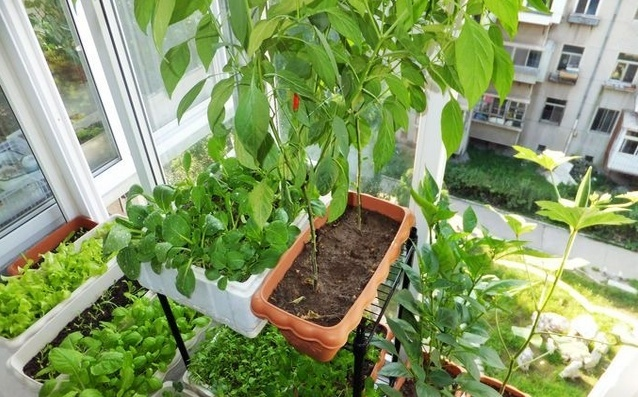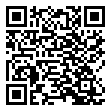|

# 阳台种花风水知识详解

随着社会的发展，大都市里人人都看累了钢筋水泥，都想看看绿色植物，特别是现在的工作都离不开电脑，天天上班对着电脑，回到家想看看绿色植物。在空旷的阳台晾晒衣服外，种些花花草草，绿绿茵茵，花香扑鼻，为生活增添了很多健康放松因子，也是一个修心养性的好习惯。而且阳台养花也有风水讲究的哦！阳台种花风水

一、阳台种花风水之阳台朝向

朝南的阳台：由于光照充足，温度较高，适合养和布置一些对光照要求较高的花卉，如彩叶草，苏铁,大花美人蕉，向日葵等。由于不同季节花卉对光照的要求不同，朝南的阳台上一年四季可培养不同的花卉。春秋温度适合时，可以培养大部分花卉。夏季，温度较高，大部分花卉都不适合养在朝南的阳台上，应该注意遮阴和降温。如没有遮阴和降温，可养不怕高温的植物花卉。如三角花，睡莲，荷花，鸡冠花，向日葵，万寿菊等。冬季，温度不是太低，如果封闭的阳台温度更高，有光照，适合养兰花，君子兰等大部分怕冷的观叶植物。但冬季夜晚温度较冷，注意防冻。

朝西的阳台：朝西的阳台由于下午光线好，温度较高，特别适合冬季养花。春秋适合养喜光花卉。但夏季温度高，光照强，只适合样耐高温日晒的花卉。

阳台种花风水

朝东的阳台：朝东的阳台由于只有上午的光线强，适合样一些怕强光照的植物，如发财树，棕竹，巴西木等。在早春和秋天，冬天也适合养阴生的花卉，如兰花，凤梨等。但冬季太冷，光照不强，只适合样不太怕冷的花卉如橡皮树，棕竹等，也可养冬季休眠的花卉。

朝北的阳台：朝北的阳台一年四季没有强烈的光照，只适合养耐阴的花卉，如鹅掌柴，绿萝，长春藤，一叶兰等。冬季没有光照而且温度低应该注意保温，或把植物花卉移到房间和有光照的阳台。

顶楼阳台：由于顶楼位置高，光照充足，温度条件好，适合样大部分的花卉。但夏季顶楼温度教楼下阳台温度高，古只能培养一些喜高温光照的花卉，如睡莲，晚莲，石榴，苏铁，向日葵等。否则，应该降温和遮阴。另外，顶楼风较大，空气流通较好，花卉生病的概率低。对大部分的花卉来说，风大易造成空气湿度降低，观叶植物及一些对空气湿度要求高的花卉，如西瓜皮椒草等，易出现叶片失水卷曲，叶缘枯焦等现象。所以一定要注意补充空气湿度。在四周多喷点水。冬季养花一定要保证温度，夜间应该移植室内，防止受冻。阳台种花风水

二、阳台种花风水禁忌

一忌：多养散发浓郁香味和刺激性气息的花卉。如兰花、玫瑰、月季、百合花、夜来香等都能散发出浓烈的香气。一盆在室，芬芳四溢，但室内假如摆放香型花卉过多，香味过浓，则会引起人的神经兴奋，特别是人在封闭阳台内长时间闻之，会引起失眠。圣诞花、万年轻散发的气体对人不利；郁金香、洋绣球散发的微粒接触过久，皮肤会过敏、发痒。

二忌：摆放数目过多。夜间大多数花卉会释放二氧化碳，吸收氧气，与人“争气”。而阳台大多封锁，空气与外界不够畅通流畅。假如室内摆放花卉过多，会减少夜间室内氧气的浓度，影响室内空气的质量，如胸闷、频发噩梦等。

三忌：室内摆放有毒性的花卉。如夹竹桃，在春、夏、秋三季其茎、叶乃至花朵都有毒，它分泌的乳白色汁液含有一种夹竹桃苷，误食会中毒；水仙花的鳞茎中含有拉丁可毒素，假如小孩误食后会引起呕吐等症状，叶和花的汁液使皮肤红肿，若汁液误入眼中，会使眼睛受害；含羞草接触过多易引起眉毛稀疏、毛发变黄，严峻时引起毛发脱落等。

四忌：阳台养花特别是未封闭的阳台及延伸架上养花要注意安全问题和邻里关系。前者特别要注意安装防护框架防止刮风及操作不慎而使花盆坠落，砸伤路人。后者防止浇水花盆漏水和掉土弄脏楼下住房的衣服，影响邻里关系。应该在花盆下放塑料底盆和安放马口铁皮或塑料板。阳台种花风水

三、阳台植物搅乱一屋风水

阳台作为住宅与外界交流重要的空间，是住宅纳气的重要通道，不容忽视。许多人喜欢在阳台堆放杂物、放洗衣机等，这样不仅会影响家居空间的美观、舒适，还会破坏家庭的运程。专家表示，最好不要在阳台堆积杂物，并且要经常进行清洁，尽量保持开阔明亮，在阳台种植物和晾衣物注意都不要将光线遮挡。对于将洗衣机放在阳台的家庭，要注意两个方位不能放，今年正西方犯三煞，洗衣机放在这个方位容易有意外、是非和疾病；东北方放洗衣机会影响家人肠胃和小孩学业，在洗衣机旁挂五帝钱可化解。另外，阳台的排水口不能开在西南、正东、东南的财位上，会漏财，开在正西、东北的凶位上反而有利。由于阳台是家中对外联系的一个重要气口，因此，也要注意回避或消除来自内外环境的不良影响。对内，阳台最好不要正对大门和厨房门，这种堪舆学上所谓的“穿心”的不良格局，容易财来财去、不聚人气。最好是能做一个玄关柜，以阻隔在大门和阳台之间；或是在阳台和厨房之间的动线上，以不影响家人行动为原则，放上柜子或屏风作为遮掩。如果空间不适合做玄关，可将阳台落地门的窗帘长时间拉上，也能起到隔断作用。对外，如果阳台外有道路直冲、屋角、尖塔等冲射，或是正对医院、庙宇等，需要采取措施来化解，常见的有八卦镜、狮子、貔貅、铜龟等化煞吉祥物，专家特别提醒，若阳台对面近距离有住宅，吉祥物使用不当就会造成“斗风水”的情况，于己于人都很不利。这时，可在阳台种植有化煞作用的植物，如仙人掌、龙骨、玉麒麟、玫瑰、杜鹃等，这类植物多干茎或花叶有刺，以冲顶不吉之气，起化煞镇宅的作用。

`声明：本文由入驻焦点开放平台的作者撰写，除焦点官方账号外，观点仅代表作者本人，不代表焦点立场错误信息举报电话： 400-099-0099，邮箱：jubao@vip.sohu.com，或点此进行意见反馈，或点此进行举报投诉。`A B C D E F G H J K L M N P Q R S T W X Y Z
A - B - C - D - E
• A
• 鞍山
• 安庆
• 安阳
• 安顺
• 安康
• 澳门
• B
• 北京
• 保定
• 包头
• 巴彦淖尔
• 本溪
• 蚌埠
• 亳州
• 滨州
• 北海
• 百色
• 巴中
• 毕节
• 保山
• 宝鸡
• 白银
• 巴州
• C
• 承德
• 沧州
• 长治
• 赤峰
• 朝阳
• 长春
• 常州
• 滁州
• 池州
• 长沙
• 常德
• 郴州
• 潮州
• 崇左
• 重庆
• 成都
• 楚雄
• 昌都
• 慈溪
• 常熟
• D
• 大同
• 大连
• 丹东
• 大庆
• 东营
• 德州
• 东莞
• 德阳
• 达州
• 大理
• 德宏
• 定西
• 儋州
• 东平
• E
• 鄂尔多斯
• 鄂州
• 恩施
F - G - H - I - J
• F
• 抚顺
• 阜新
• 阜阳
• 福州
• 抚州
• 佛山
• 防城港
• G
• 赣州
• 广州
• 桂林
• 贵港
• 广元
• 广安
• 贵阳
• 固原
• H
• 邯郸
• 衡水
• 呼和浩特
• 呼伦贝尔
• 葫芦岛
• 哈尔滨
• 黑河
• 淮安
• 杭州
• 湖州
• 合肥
• 淮南
• 淮北
• 黄山
• 菏泽
• 鹤壁
• 黄石
• 黄冈
• 衡阳
• 怀化
• 惠州
• 河源
• 贺州
• 河池
• 海口
• 红河
• 汉中
• 海东
• 怀来
• I
• J
• 晋中
• 锦州
• 吉林
• 鸡西
• 佳木斯
• 嘉兴
• 金华
• 景德镇
• 九江
• 吉安
• 济南
• 济宁
• 焦作
• 荆门
• 荆州
• 江门
• 揭阳
• 金昌
• 酒泉
• 嘉峪关
K - L - M - N - P
• K
• 开封
• 昆明
• 昆山
• L
• 廊坊
• 临汾
• 辽阳
• 连云港
• 丽水
• 六安
• 龙岩
• 莱芜
• 临沂
• 聊城
• 洛阳
• 漯河
• 娄底
• 柳州
• 来宾
• 泸州
• 乐山
• 六盘水
• 丽江
• 临沧
• 拉萨
• 林芝
• 兰州
• 陇南
• M
• 牡丹江
• 马鞍山
• 茂名
• 梅州
• 绵阳
• 眉山
• N
• 南京
• 南通
• 宁波
• 南平
• 宁德
• 南昌
• 南阳
• 南宁
• 内江
• 南充
• P
• 盘锦
• 莆田
• 平顶山
• 濮阳
• 攀枝花
• 普洱
• 平凉
Q - R - S - T - W
• Q
• 秦皇岛
• 齐齐哈尔
• 衢州
• 泉州
• 青岛
• 清远
• 钦州
• 黔南
• 曲靖
• 庆阳
• R
• 日照
• 日喀则
• S
• 石家庄
• 沈阳
• 双鸭山
• 绥化
• 上海
• 苏州
• 宿迁
• 绍兴
• 宿州
• 三明
• 上饶
• 三门峡
• 商丘
• 十堰
• 随州
• 邵阳
• 韶关
• 深圳
• 汕头
• 汕尾
• 三亚
• 三沙
• 遂宁
• 山南
• 商洛
• 石嘴山
• T
• 天津
• 唐山
• 太原
• 通辽
• 铁岭
• 泰州
• 台州
• 铜陵
• 泰安
• 铜仁
• 铜川
• 天水
• 天门
• W
• 乌海
• 乌兰察布
• 无锡
• 温州
• 芜湖
• 潍坊
• 威海
• 武汉
• 梧州
• 渭南
• 武威
• 吴忠
• 乌鲁木齐
X - Y - Z
• X
• 邢台
• 徐州
• 宣城
• 厦门
• 新乡
• 许昌
• 信阳
• 襄阳
• 孝感
• 咸宁
• 湘潭
• 湘西
• 西双版纳
• 西安
• 咸阳
• 西宁
• 仙桃
• 西昌
• Y
• 运城
• 营口
• 盐城
• 扬州
• 鹰潭
• 宜春
• 烟台
• 宜昌
• 岳阳
• 益阳
• 永州
• 阳江
• 云浮
• 玉林
• 宜宾
• 雅安
• 玉溪
• 延安
• 榆林
• 银川
• Z
• 张家口
• 镇江
• 舟山
• 漳州
• 淄博
• 枣庄
• 郑州
• 周口
• 驻马店
• 株洲
• 张家界
• 珠海
• 湛江
• 肇庆
• 中山
• 自贡
• 资阳
• 遵义
• 昭通
• 张掖
• 中卫

1室1厅1厨1卫1阳台

1
2
3
4
5

0
1
2

1

1

0
1
2
3报名成功，资料已提交审核A B C D E F G H J K L M N P Q R S T W X Y Z
A - B - C - D - E
• A
• 鞍山
• 安庆
• 安阳
• 安顺
• 安康
• 澳门
• B
• 北京
• 保定
• 包头
• 巴彦淖尔
• 本溪
• 蚌埠
• 亳州
• 滨州
• 北海
• 百色
• 巴中
• 毕节
• 保山
• 宝鸡
• 白银
• 巴州
• C
• 承德
• 沧州
• 长治
• 赤峰
• 朝阳
• 长春
• 常州
• 滁州
• 池州
• 长沙
• 常德
• 郴州
• 潮州
• 崇左
• 重庆
• 成都
• 楚雄
• 昌都
• 慈溪
• 常熟
• D
• 大同
• 大连
• 丹东
• 大庆
• 东营
• 德州
• 东莞
• 德阳
• 达州
• 大理
• 德宏
• 定西
• 儋州
• 东平
• E
• 鄂尔多斯
• 鄂州
• 恩施
F - G - H - I - J
• F
• 抚顺
• 阜新
• 阜阳
• 福州
• 抚州
• 佛山
• 防城港
• G
• 赣州
• 广州
• 桂林
• 贵港
• 广元
• 广安
• 贵阳
• 固原
• H
• 邯郸
• 衡水
• 呼和浩特
• 呼伦贝尔
• 葫芦岛
• 哈尔滨
• 黑河
• 淮安
• 杭州
• 湖州
• 合肥
• 淮南
• 淮北
• 黄山
• 菏泽
• 鹤壁
• 黄石
• 黄冈
• 衡阳
• 怀化
• 惠州
• 河源
• 贺州
• 河池
• 海口
• 红河
• 汉中
• 海东
• 怀来
• I
• J
• 晋中
• 锦州
• 吉林
• 鸡西
• 佳木斯
• 嘉兴
• 金华
• 景德镇
• 九江
• 吉安
• 济南
• 济宁
• 焦作
• 荆门
• 荆州
• 江门
• 揭阳
• 金昌
• 酒泉
• 嘉峪关
K - L - M - N - P
• K
• 开封
• 昆明
• 昆山
• L
• 廊坊
• 临汾
• 辽阳
• 连云港
• 丽水
• 六安
• 龙岩
• 莱芜
• 临沂
• 聊城
• 洛阳
• 漯河
• 娄底
• 柳州
• 来宾
• 泸州
• 乐山
• 六盘水
• 丽江
• 临沧
• 拉萨
• 林芝
• 兰州
• 陇南
• M
• 牡丹江
• 马鞍山
• 茂名
• 梅州
• 绵阳
• 眉山
• N
• 南京
• 南通
• 宁波
• 南平
• 宁德
• 南昌
• 南阳
• 南宁
• 内江
• 南充
• P
• 盘锦
• 莆田
• 平顶山
• 濮阳
• 攀枝花
• 普洱
• 平凉
Q - R - S - T - W
• Q
• 秦皇岛
• 齐齐哈尔
• 衢州
• 泉州
• 青岛
• 清远
• 钦州
• 黔南
• 曲靖
• 庆阳
• R
• 日照
• 日喀则
• S
• 石家庄
• 沈阳
• 双鸭山
• 绥化
• 上海
• 苏州
• 宿迁
• 绍兴
• 宿州
• 三明
• 上饶
• 三门峡
• 商丘
• 十堰
• 随州
• 邵阳
• 韶关
• 深圳
• 汕头
• 汕尾
• 三亚
• 三沙
• 遂宁
• 山南
• 商洛
• 石嘴山
• T
• 天津
• 唐山
• 太原
• 通辽
• 铁岭
• 泰州
• 台州
• 铜陵
• 泰安
• 铜仁
• 铜川
• 天水
• 天门
• W
• 乌海
• 乌兰察布
• 无锡
• 温州
• 芜湖
• 潍坊
• 威海
• 武汉
• 梧州
• 渭南
• 武威
• 吴忠
• 乌鲁木齐
X - Y - Z
• X
• 邢台
• 徐州
• 宣城
• 厦门
• 新乡
• 许昌
• 信阳
• 襄阳
• 孝感
• 咸宁
• 湘潭
• 湘西
• 西双版纳
• 西安
• 咸阳
• 西宁
• 仙桃
• 西昌
• Y
• 运城
• 营口
• 盐城
• 扬州
• 鹰潭
• 宜春
• 烟台
• 宜昌
• 岳阳
• 益阳
• 永州
• 阳江
• 云浮
• 玉林
• 宜宾
• 雅安
• 玉溪
• 延安
• 榆林
• 银川
• Z
• 张家口
• 镇江
• 舟山
• 漳州
• 淄博
• 枣庄
• 郑州
• 周口
• 驻马店
• 株洲
• 张家界
• 珠海
• 湛江
• 肇庆
• 中山
• 自贡
• 资阳
• 遵义
• 昭通
• 张掖
• 中卫• 手机• 分享
• 设计
免费设计
• 计算器
装修计算器
• 入驻
合作入驻
• 联系
联系我们
• 置顶
返回顶部